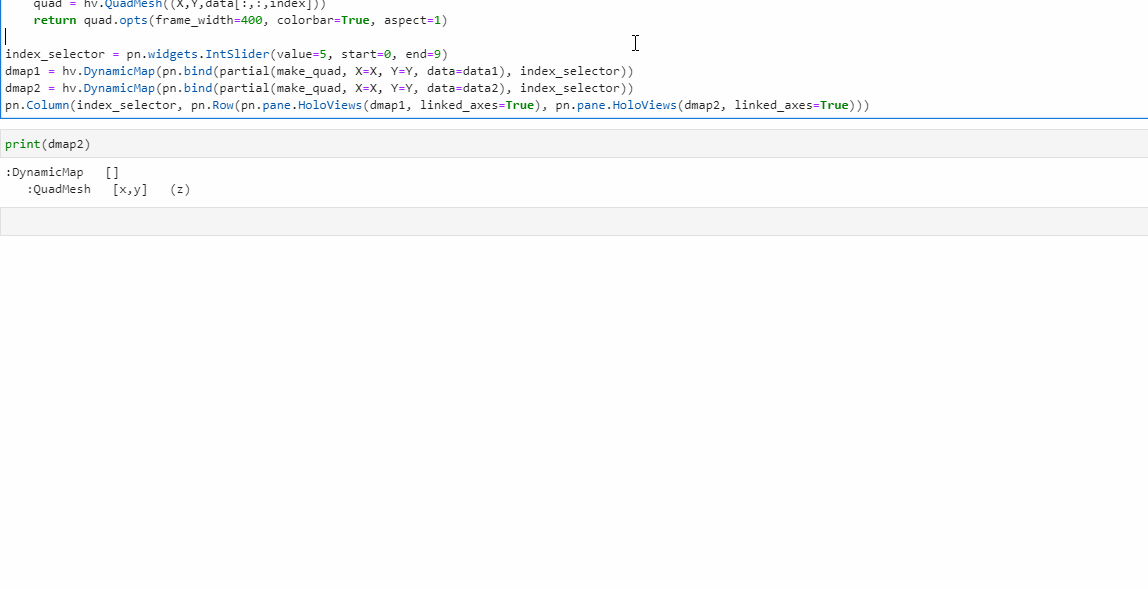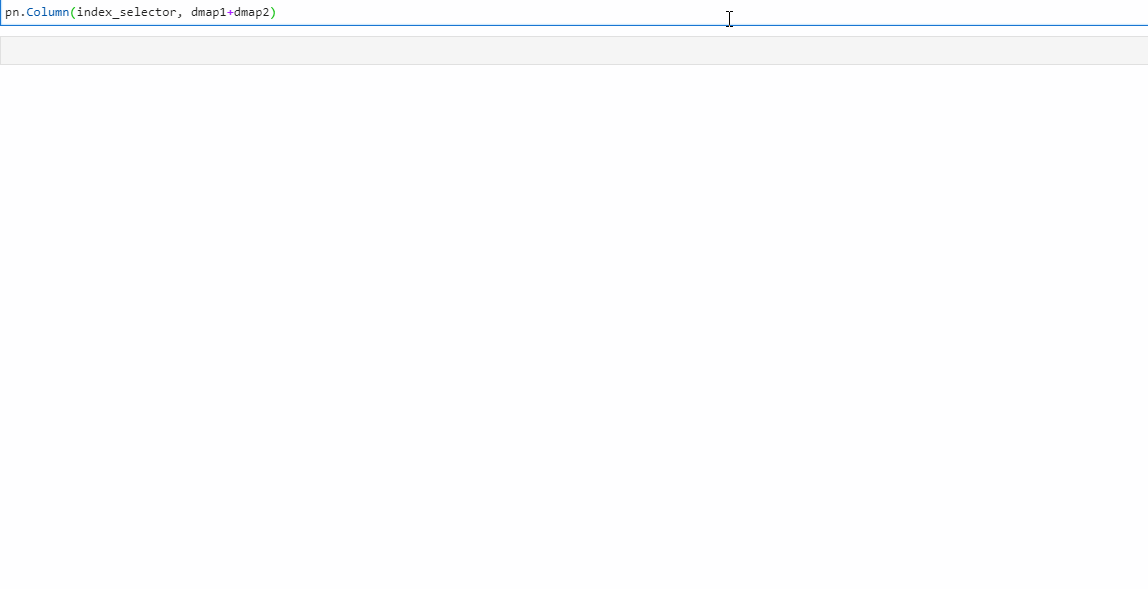# Link z axis of holoviews plot inside panel

Hello,

I have a problem to share the z axis of dynamic QuadMesh inside a panel
Here is an example:

``````import panel as pn
import numpy as np
import holoviews as hv
from functools import partial
hv.extension("bokeh")
pn.extension()

theta = np.r_[np.linspace(0, 2*np.pi, 100)]
data1 = np.random.rand(*X.shape, 10)
data2 = np.random.rand(*X.shape, 10) * (np.arange(10)+1)[None, None,:]

index_selector = pn.widgets.IntSlider(value=5, start=0, end=9)
dmap1 = hv.DynamicMap(pn.bind(partial(make_quad, X=X, Y=Y, data=data1), index_selector))
dmap2 = hv.DynamicMap(pn.bind(partial(make_quad, X=X, Y=Y, data=data2), index_selector))
``````I’d like both quadmesh share the same colorbar
I tried to use a hv Layout for both dynamicmaps
`pn.Column(index_selector, dmap1 + dmap2)`
but it works only on the first render, if the value of the slider is changed colorbar are not shared anymoreI could get it to work with the following:

``````import panel as pn
import numpy as np
import holoviews as hv
from functools import partial
hv.extension("bokeh")
pn.extension()

theta = np.r_[np.linspace(0, 2*np.pi, 100)]
data1 = np.random.rand(*X.shape, 10)
data2 = np.random.rand(*X.shape, 10) * (np.arange(10)+1)[None, None,:]

index_selector = pn.widgets.IntSlider(value=5, start=0, end=9)
``````

But if I change the last line to `pn.Column(index_selector, hv.DynamicMap(pn.bind(make_quads, index_selector)))` it breaks the colorbar sync.

Hope this helps.

Actually I’d like to have the DynamicMaps since it’s more reactive, and I’d like to be able to use panel layout to put results in different tabs

my solution would be:

``````import param
import panel as pn
import numpy as np
import holoviews as hv
from functools import partial
hv.extension("bokeh")
pn.extension()

theta = np.r_[np.linspace(0, 2*np.pi, 100)]
data1 = np.random.rand(*X.shape, 10)
data2 = np.random.rand(*X.shape, 10) * (np.arange(10)+1)[None, None,:]

index_selector = pn.widgets.IntSlider(value=5, start=0, end=9)
clim = pn.widgets.RangeSlider()
@pn.depends(index_selector, watch=True)
def update_clim(index):
d = np.stack((data1[:,:,index], data2[:,:,index]))
cmin, cmax = d.min(), d.max()
with param.batch_watch(clim):
clim.start = cmin
clim.end = cmax
clim.value = (cmin, cmax)
clim.step = (cmax-cmin)/100
update_clim(index_selector.value)
dmap1 = hv.DynamicMap(pn.bind(partial(make_quad, X=X, Y=Y, data=data1), index_selector)).apply.opts(clim=clim.param.value)
dmap2 = hv.DynamicMap(pn.bind(partial(make_quad, X=X, Y=Y, data=data2), index_selector)).apply.opts(clim=clim.param.value)
pn.Column(index_selector, clim, pn.Row(pn.pane.HoloViews(dmap1), pn.pane.HoloViews(dmap2)))
``````

in the same time I can adjust the z axis but I see this more like a workaround than the expected solution

@xavArtley Could you file a Panel issue? We should investigate actually reusing the same color mapper instances.

1 Like

I properly wasn’t that clear in my last post, but what I wanted to say is it works as you would expect without DynamicMap but breaks the colorbar sync when you add it. So it is highly likely a more advanced layout with separate plots and in taps will still not work.